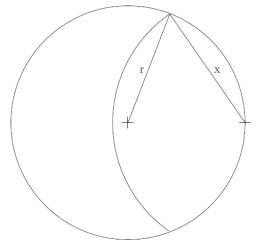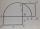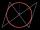# Goat and circles

What is the radius of a circle centered on the other circle and the intersection of the two circles is equal to half the area of the first circle?

This task is the mathematical expression of the role of agriculture. The farmer has circular land on which graze goat. Because the farmer wants to spend the grass throughout the two days, tie it to a stake on the edge of the circle and length of rope that for the first half of grass. Second day give they a whole circle, where they can feed off the remaining one-half of grass.

Correct result:

p=x/r =  116.9 %

#### Solution:

$S_1 + S_2 = \dfrac12 S \ \\ S = \pi r^2 \ \\ S_1 = \dfrac12x^2(\alpha -\sin \alpha) \ \\ S_2 = \dfrac12r^2((2\pi - 2\alpha) -\sin (2\pi - 2\alpha)) \ \\ \ \\ x = 2r \cos (\alpha/2) \ \\ \ \\ \ \\ \alpha = \tan \alpha - \dfrac{ \pi }{ 2 \cos \alpha } \ \\ \ \\ \alpha_1 = 1.9056957293099 = 66^\circ 23'25" \ \\ p_1 = x/r = 1.1587284730181 = 115.87 \% \ \\ \alpha_2 = 4.0903294575997 = -52^\circ 20'34" \ \\ p_2 = x/r = -0.91355336099303 = -91.36 \% \ \\ \alpha_3 = 7.9263876105649 = -78^\circ 2'33" \ \\ p_3 = x/r = -1.3620993158089 = -136.21 \% \ \\ \alpha_4 = 10.756961303274 = 70^\circ 48'29" \ \\ p_4 = x/r = 1.235835638602 = 123.58 \% \ \\ \alpha_5 = -11.0473869356 = 83^\circ 6'1" \ \\ p_5 = x/r = 1.4503719997774 = 145.04 \% \ \\ \alpha_6 = -7.5128894066161 = -93^\circ 36'18" \ \\ p_6 = x/r = -1.6337175982743 = -163.37 \% \ \\ \alpha_7 = -4.8322830401789 = -85^\circ 44'15" \ \\ p_7 = x/r = -1.49640036639 = -149.64 \% \ \\ \ \\ \ \\$We would be pleased if you find an error in the word problem, spelling mistakes, or inaccuracies and send it to us. Thank you!Tips to related online calculators
Do you have a linear equation or system of equations and looking for its solution? Or do you have quadratic equation?
Most natural application of trigonometry and trigonometric functions is a calculation of the triangles. Common and less common calculations of different types of triangles offers our triangle calculator. Word trigonometry comes from Greek and literally means triangle calculation.

#### You need to know the following knowledge to solve this word math problem:

We encourage you to watch this tutorial video on this math problem:

## Next similar math problems:

• Isosceles IVIn an isosceles triangle ABC is |AC| = |BC| = 13 and |AB| = 10. Calculate the radius of the inscribed (r) and described (R) circle.
• Tractor wheelsThe front wheel of the tractor has a circumference of 18 dm and the rear 60 dm. We will make a red mark on the lowest point of both wheels. The tractor then starts. At what distance from the start will both marks appear identically at the bottom again?
• Same areaThere is a given triangle. Construct a square of the same area.
• Circle in rhombusIn the rhombus is inscribed circle. Contact points of touch divide the sides to parts of length 19 cm and 6 cm. Calculate the circle area.
• RhombusIt is given a rhombus of side length a = 19 cm. Touch points of inscribed circle divided his sides into sections a1 = 5 cm and a2 = 14 cm. Calculate the radius r of the circle and the length of the diagonals of the rhombus.
• GearsThe front gear on the bike has 32 teeth and the rear, on the wheel, has 12 teeth. How many times does the rear wheel of the bike turns if you turn the right pedal 30 times? What distance will you go if the circumference of the bicycle wheel is 250 cm?
• Right triangle - ratioThe lengths of the legs of the right triangle ABC are in ratio b = 2: 3. The hypotenuse is 10 cm long. Calculate the lengths of the legs of that triangle.
• DodecagonCalculate the size of the smaller of the angles determined by lines A1 A4 and A2 A10 in the regular dodecagon A1A2A3. .. A12. Express the result in degrees.
• Find the 13Find the equation of the circle inscribed in the rhombus ABCD where A[1, -2], B[8, -3] and C[9, 4].
• RT sidesFind the sides of a rectangular triangle if legs a + b = 17cm and the radius of the written circle ρ = 2cm.Calculate the content of a regular 15-sides polygon inscribed in a circle with radius r = 4. Express the result to two decimal places.In the circle with a radius 7.5 cm are constructed two parallel chord whose lengths are 9 cm and 12 cm. Calculate the distance of these chords (if there are two possible solutions write both).To circle with a radius of 41 cm from the point R guided two tangents. The distance of both points of contact is 16 cm. Calculate the distance from point R and circle centre.A triangle has a hypotenuse of 55 and an altitude to the hypotenuse of 33. What is the area of the triangle?An ABCD square with a side length of 100 mm is given. Calculate the radius of the circle that passes through the vertices B, C and the center of the side AD.It is given a rhombus with side a = 6 cm and the radius of the inscribed circle r = 2 cm. Calculate the length of its two diagonals.A sphere is inscribed in the cone (the intersection of their boundaries consists of a circle and one point). The ratio of the surface of the ball and the contents of the base is 4: 3. A plane passing through the axis of a cone cuts the cone in an isoscele